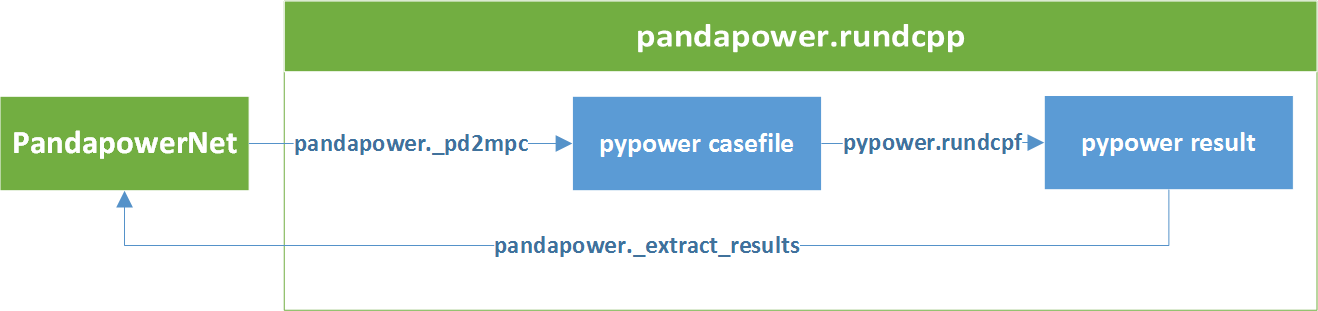# DC Power flow¶

Warning

To run an AC power flow with DC power flow initialization, use the AC power flow with init=”dc”.

pandapower uses PYPOWER to solve the DC power flow problem:`pandapower.``rundcpp`(net, trafo_model='t', trafo_loading='current', recycle=None, check_connectivity=True, switch_rx_ratio=2, trafo3w_losses='hv', **kwargs)

Runs PANDAPOWER DC Flow

INPUT:

net - The pandapower format network

OPTIONAL:

trafo_model (str, “t”) - transformer equivalent circuit model pandapower provides two equivalent circuit models for the transformer:

• “t” - transformer is modeled as equivalent with the T-model. This is consistent with PowerFactory and is also more accurate than the PI-model. We recommend using this transformer model.

• “pi” - transformer is modeled as equivalent PI-model. This is consistent with Sincal, but the method is questionable since the transformer is physically T-shaped. We therefore recommend the use of the T-model.

trafo_loading (str, “current”) - mode of calculation for transformer loading

Transformer loading can be calculated relative to the rated current or the rated power. In both cases the overall transformer loading is defined as the maximum loading on the two sides of the transformer.

• “current”- transformer loading is given as ratio of current flow and rated current of the transformer. This is the recommended setting, since thermal as well as magnetic effects in the transformer depend on the current.

• “power” - transformer loading is given as ratio of apparent power flow to the rated apparent power of the transformer.

check_connectivity (bool, False) - Perform an extra connectivity test after the conversion from pandapower to PYPOWER

If true, an extra connectivity test based on SciPy Compressed Sparse Graph Routines is perfomed. If check finds unsupplied buses, they are put out of service in the PYPOWER matrix

switch_rx_ratio (float, 2) - rx_ratio of bus-bus-switches. If impedance is zero, buses connected by a closed bus-bus switch are fused to model an ideal bus. Otherwise, they are modelled as branches with resistance defined as z_ohm column in switch table and this parameter

trafo3w_losses (str, “hv”) - defines where open loop losses of three-winding transformers are considered. Valid options are “hv”, “mv”, “lv” for HV/MV/LV side or “star” for the star point.

**kwargs - options to use for PYPOWER.runpf

Note

If you are interested in the pypower casefile that pandapower is using for power flow, you can find it in net[“_ppc”]. However all necessary informations are written into the pandpower format net, so the pandapower user should not usually have to deal with pypower.